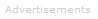# Definition of Frequency

What is Frequency?

Frequency is the number of events per unit of time where an event is repeating at regularly spaced time intervals.

Frequency's units are per second, written s-1 or hertz, Hz. Hertz and per second are identical units, i.e. 1 s-1 = 1 Hz.Example 1
When describing a moving wave, frequency means the number of peaks which pass a stationary point in a given amount of time.

Example 2
When describing a heartbeat, frequency means the number of beats per second.

Example 3
When describing a rotating wheel, frequency means the number times the wheel rotates through 360° per second.

Worked Example 1
A fast drummer beats a drum 150 times in 30 seconds keeping a regular rhythm. What is the frequency of the beats?

frequency   =   (number of beats) / time   =   150/30s   = 5   s-1 or 5 Hz

Worked Example 2
A humming bird's wings go up and down again 440 times in 8 seconds. What is the frequency of the wing beats?

frequency   =   (number of beats) / time   =   440/8s   =   55 Hz

Worked Example 3
On the beach, you count waves as they pass a rock. You notice that 6 wave peaks pass the rock in a minute. What is the frequency of the waves?

frequency   =   (number of wave peaks) / time   =   6/60s   =   0.1 Hz
Frequency and Period

Frequency is the inverse of period, T. The period is the time taken between events that are repeating at regular intervals.

f = 1/T

Worked Example 4
The frequency of a fan's rotation is quoted as 100 Hz. How long does a complete 360° rotation of the fan take?

f = 1/T
⇒ T = 1/f
⇒ T = 1/100 s-1
⇒ T = 0.01 sSearch the Dictionary for More Terms